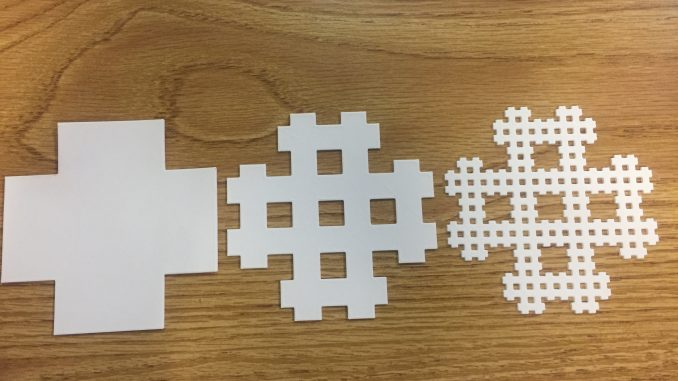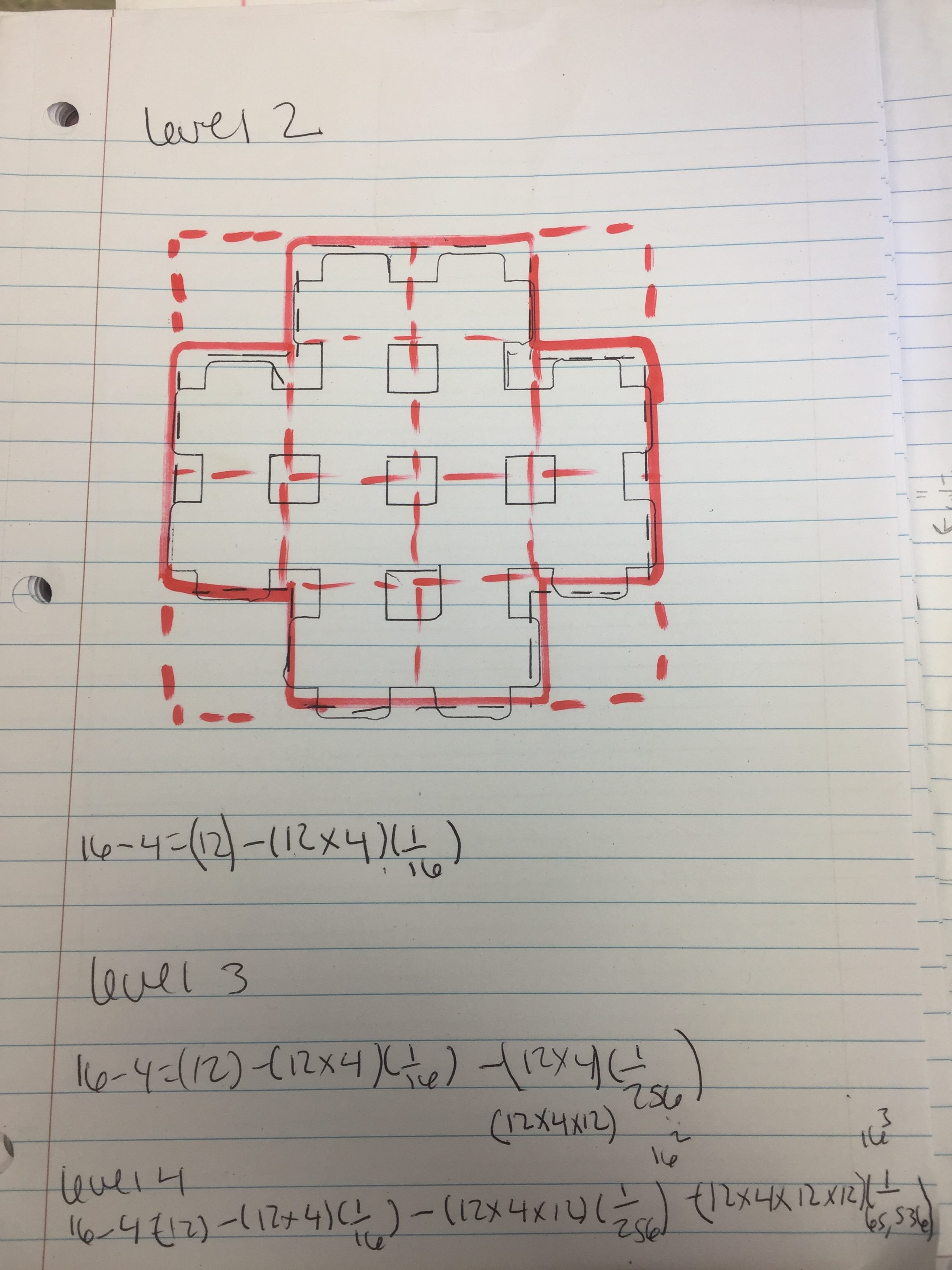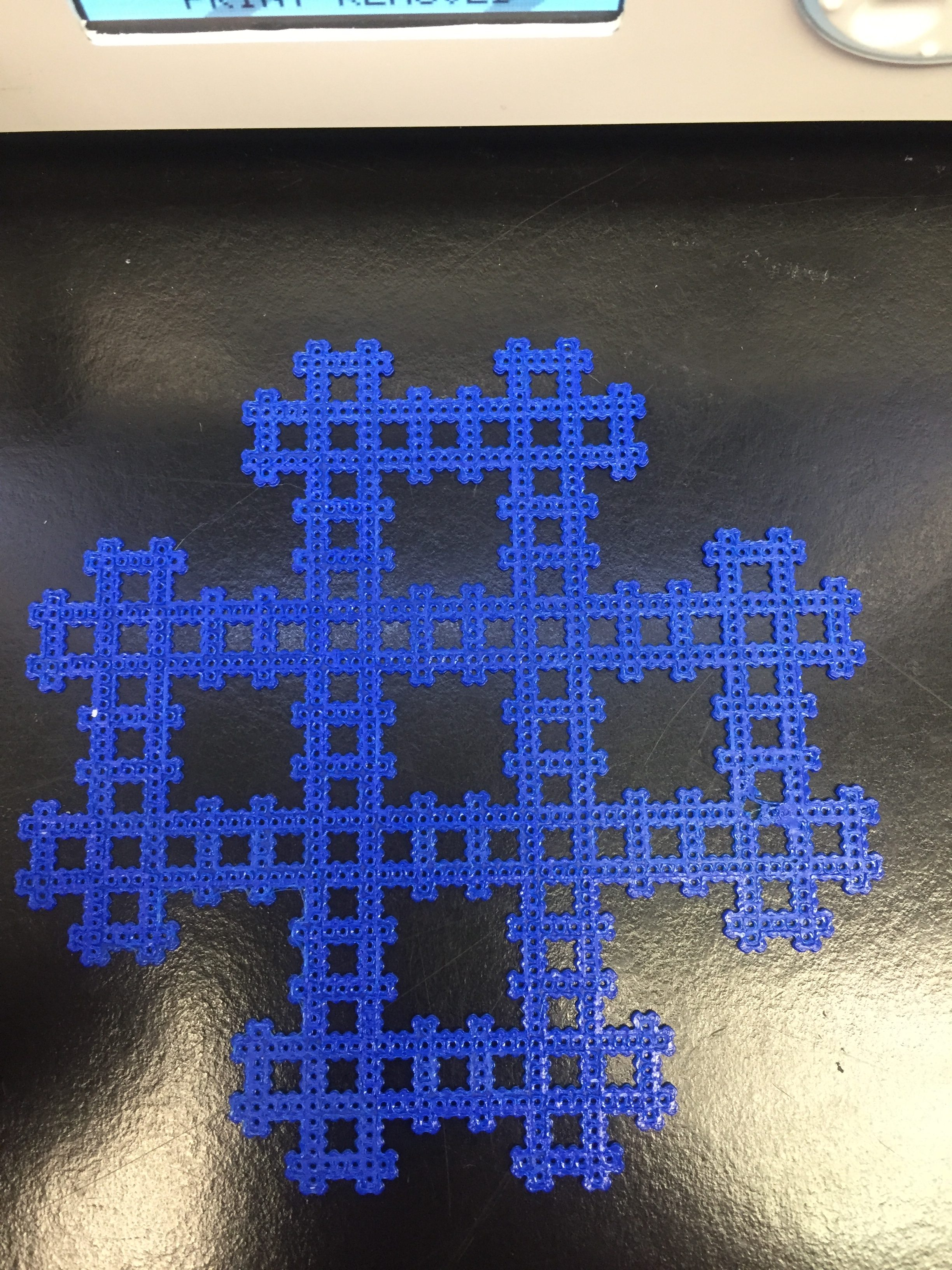# Four Corner Fractal CarpetTo make my fractal I removed the four corners of the blank carpet. I removed the coordinates [0,0], [3,0], [0,3], and [3,3]. With each iteration the  four corners of each square get removed. I designed my fractal this way because I wanted something neat and orderly looking. I liked the cross shape of the first iteration so I went with it.I also was able to create a level 4 of the Four Corner Fractal:level 1: (16-4)-(12×4)(1/16)

### Infinite Geometric Series Calculations

The infinite calculation of the fractal carpet if calculated on and on would end up with 0 because as you remove more and more squares from the fractal, eventually you run out of fractal.

Infinite series: 16 – [(4×1)+(4×12)(1/16)+(4)(12/16^2)+(4)(12/16^3)…∞]

### Dimension Calculation

To get the dimension of the fractal carpet each iteration is 1/4 the size of the one that came before it. When doing the calculation once you get 12 you plug it into the logarithm log base A of B, which gets you log base 4 of twelve and then you get dimension.

S^D=1/the number of copies

(1/4)^D=1/12

1/(4^D)=1/12

4^D=12

log4(12)=D

D=1.792